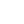# Advent of Code 2022 - Day 4 Javascript Solution

By Henri ParviainenIt's 4th of December and that means it's time to do the Advent of Code challenge for the day. This time there is a mix up in the cleaning arrangements and the elves need our help to sort it out. Here is my Javascript solution for day 4.

## Disclaimer

This solution is not the fastest implementation nor is it claiming to be the best way to solve the problem. There are many ways to solve it and this is just how I did it with Javascript. By sharing I hope it can help someone who is struggling to find a solution to the puzzle.

## Solution

To be able to run my solution, you should have same project setup as me.

First, download the input and save it as a `txt` file in the same directory as you have your javascript files. If you don't yet have any javascript files - make two - one for each part.

So at the starting point, your folder structure should be:

``````/
-- part1.js
-- part2.js
-- input.txt``````

### Part 1

Add the following code to your `part1.js` file.

``````let fs = require("fs");

let assignmentPairs = input.split("\n");

assignmentPairs = assignmentPairs.map(assignmentPair =>
assignmentPair.split(",")
);

let fullyContainsAmount = 0;

for (const assignmentPair of assignmentPairs) {
let assignment1start = +assignmentPair.split("-");
let assignment1end = +assignmentPair.split("-");

let assignment2start = +assignmentPair.split("-");
let assignment2end = +assignmentPair.split("-");

// Check if first fully contains the second

if (
assignment1start <= assignment2start &&
assignment1end >= assignment2end
) {
fullyContainsAmount += 1;
console.log(
`assignment 1 (\${assignment1start} - \${assignment1end}) fully contains assignment 2 (\${assignment2start}- \${assignment2end})`
);
}

// Check if second fully contains the first
else if (
assignment2start <= assignment1start &&
assignment2end >= assignment1end
) {
fullyContainsAmount += 1;
console.log(
`assignment 2 (\${assignment2start} - \${assignment2end}) fully contains assignment 1 (\${assignment1start}- \${assignment1end})`
);
}
}

console.log(fullyContainsAmount);``````

Run in it with `node part1.js` and you'll have your answer for the first part!

### Part 2

For the second part, add the following code to `part2.js`.

``````let fs = require("fs");

let assignmentPairs = input.split("\n");

console.log(assignmentPairs);

assignmentPairs = assignmentPairs.map(assignmentPair =>
assignmentPair.split(",")
);

let partialMatchesAmount = 0;

for (const assignmentPair of assignmentPairs) {
let assignment1start = +assignmentPair.split("-");
let assignment1end = +assignmentPair.split("-");

let assignment2start = +assignmentPair.split("-");
let assignment2end = +assignmentPair.split("-");

// Check if first range is fully before the second

if (
assignment1start < assignment2start &&
assignment1end < assignment2start
) {
console.log("no overlap");
}

// Check if second range is fully before the first
else if (
assignment2start < assignment1start &&
assignment2end < assignment1start
) {
console.log("no overlap");
} else {
partialMatchesAmount += 1;
}
}

console.log(partialMatchesAmount);``````

By running `node part2.js` you have the answer for the second part as well.

That's it for todays puzzles. I'll be back tomorrow with a solution for day 5!